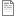# SUES_AVGLSTK

Suess glacier average stake height spreadsheet units and column descriptions

## File:SUES_AVGLSTK.csv (11.19 KB)
Variables
DATASET CODE
• Label:
• Definition: Code representing average stake heights, snow depths dataset
• Type: Nominal
• Missing values: None specified
GLACIER
• Label:
• Definition: NAME OF GLACIER WHERE MEASUREMENT WAS MADE
• Type: Nominal
• Missing values: None specified
STAKE
• Label:
• Definition: UNIQUE NUMBER FOR EACH STAKE ON GLACIER
• Type: Nominal
• Missing values: None specified
STAKE_REPLCMNT
• Label:
• Definition: Letter corresponding to original and replacement stakes (A = original, B = first replacement, C = second replacement etc.)
• Type: Nominal
• Missing values: None specified
DATE_TIME
• Label:
• Definition: DATE OF DATA MEASUREMENT
• Type: Date/time
• Date format: MM/DD/YYYY
• Missing values: None specified
SURFACE
• Label:
• Definition: Surface material to which measurement is made
• Type: Nominal
• Missing values: None specified
SNOW_DEPTH_AVG
• Label:
• Definition: Average depth of snow covering ice (calculated from 1-8 replications)
• Type: Physical quantity
• Unit: centimeter
• Maximum: 200
• Minimum: 0
• Precision: 0.1
• Missing values:
• NULL = None given
SNOW_DEPTH_STD_DEV
• Label:
• Definition: Standard deviation for measured snow depths (calculated from 1-8 replications)
• Type: Physical quantity
• Unit: centimeter
• Maximum: 10
• Minimum: 0
• Precision: 0.1
• Missing values:
• NULL = None given
SNOW_DEPTH_COMMENTS
• Label:
• Definition: Comments regarding snow depth measurements
• Type: Nominal
• Missing values: None specified
STK_HT_AVG
• Label:
• Definition: Average distance from top of stake to the glacier surface (calculated from 1-8 replications)
• Type: Physical quantity
• Unit: centimeter
• Maximum: 400
• Minimum: 0
• Precision: 0.1
• Missing values:
• NULL = None given
STK_HT_STD_DEV
• Label:
• Definition: Standard deviation for measured stake heights (calculated from 1-8 replications)
• Type: Physical quantity
• Unit: centimeter
• Maximum: 10
• Minimum: 0
• Precision: 0.1
• Missing values:
• NULL = None given
STK_HT_COMMENTS
• Label:
• Definition: Comments regarding stake height measurements
• Type: Nominal
• Missing values: None specified
GLSTKID
• Label:
• Definition: CODE FROM GLACIER / STAKE/ DATE OF COORDINATE MEASUREMENT (TO BE USED FOR GIS MAPPING)
• Type: Nominal
• Missing values: None specified
FILE NAME
• Label:
• Definition: RAW DATA FILE CONTAINING INFORMATION
• Type: Nominal
• Missing values: None specified
Data Source Details

28

Column

"

,

\n